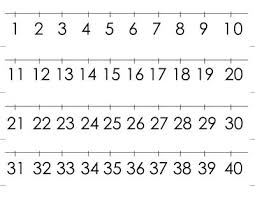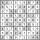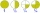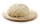# The expression

What is the value of the expression
$\frac{\left({6}^{2}+60\right)}{{2}^{3}}$

x =  12

### Step-by-step explanation:

$x=\frac{\left({6}^{2}+60\right)}{{2}^{3}}=12$Did you find an error or inaccuracy? Feel free to write us. Thank you!Tips to related online calculators
Need help to calculate sum, simplify or multiply fractions? Try our fraction calculator.

## Related math problems and questions:

• Evaluate expr with 2 varsIf a=6 and x=2, find the value of (2ax + 7x- 10) /(4ax-3a-2)
• Two numbers and its productThe product of two numbers are 2/3. If on of them is 1/10, what is the other?
• Find unknown 2Find unknown denominator: 2/3 -5/? = 1/4
• Simple equation 324 = n • 27, solve for n
• Evaluate expressionCalculate the value of the expression z/3 - 2 z/9 + 1/6, for z = 2
• ExpressionIf it is true that (l + 15 w)/(w) = 6, then the value of the expression (13 l)/(12 w) is:
• Distributive propertyVerify the distributive property a×(b+c)=(a×b)+(a*c) for the rational number a=5/8, b=7/4 and c=2/3
• Even / odd numbersa / Using variable n write two consecutive odd numbers. b / the sum of three consecutive odd numbers is 333. What are this numbers?
• The angles ratioThe angles in the ABC triangle are in the ratio 1: 2: 3. find the sizes of the angles and determine what kind of a triangle it is.
• The sum 12The sum of 3 mixed numbers is 20 13/15. two of the numbers are 6 1/3 and 7 5/6. what is the third number?
• Comparing and sortingArrange in descending order this fractions: 2/7, 7/10 & 1/2
• Product increasedWhen the product of 2/3 and 6/10 is increased by 2/5, the result is?
• The doughThe dough contains water, flour, and sugar. Water and flour in a ratio of 2: 3, flour and sugar in a 2: 1. Find the ratio of all three components of the dough.
• Simplest form of a fractionWhich one of the following fraction after reducing in simplest form is not equal to 3/2? a) 15/20 b) 12/8 c) 27/18 d) 6/4
• Numbers divisionWith what number should be divided mixed number 2 3/4 to get 11/12?
• Simplify 7Simplify. 7-1/3÷ 3-2/3 of 2+ 4-1/2÷ 2-1/4+ 1/2 solution and by step by step3 3/4 + 2 3/5 + 5 1/2 Show your solution.